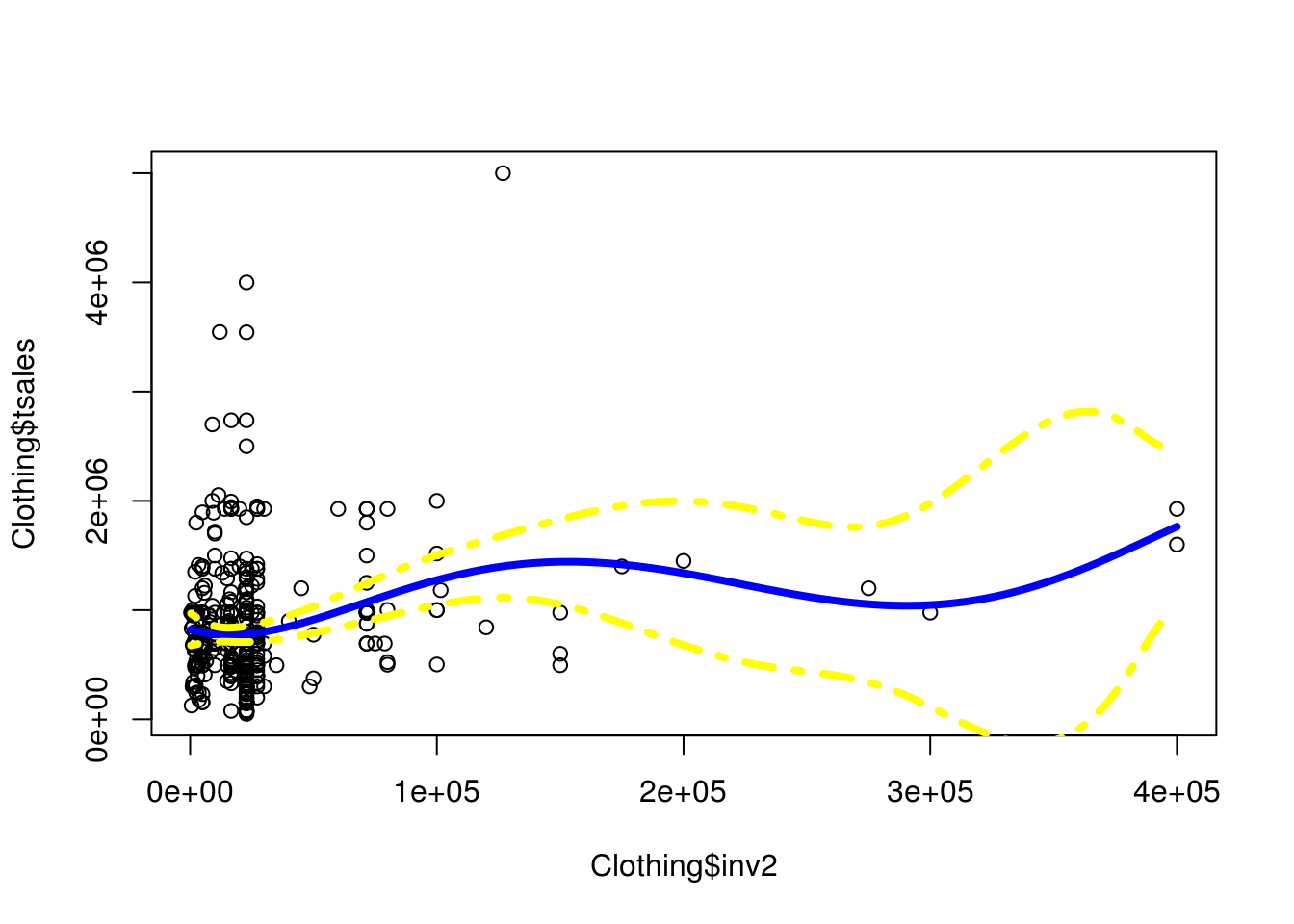# Polynomial Regression in R

Polynomial regression is one of the easiest ways to fit a non-linear line to a data set. This is done through the use of higher order polynomials such as cubic, quadratic, etc to one or more predictor variables in a model.Generally, polynomial regression is used for one predictor and one outcome variable. When there are several predictor variables it is more common to use generalized additive modeling/ In this post, we will use the “Clothing” dataset from the “Ecdat” package to predict total sales with the use of polynomial regression. Below is some initial code.

````library(Ecdat)````
data(Clothing)
str(Clothing)``````
``````## 'data.frame':    400 obs. of  13 variables:
##  \$ tsales : int  750000 1926395 1250000 694227 750000 400000 1300000 495340 1200000 495340 ...
##  \$ sales  : num  4412 4281 4167 2670 15000 ...
##  \$ margin : num  41 39 40 40 44 41 39 28 41 37 ...
##  \$ nown   : num  1 2 1 1 2 ...
##  \$ nfull  : num  1 2 2 1 1.96 ...
##  \$ npart  : num  1 3 2.22 1.28 1.28 ...
##  \$ naux   : num  1.54 1.54 1.41 1.37 1.37 ...
##  \$ hoursw : int  76 192 114 100 104 72 161 80 158 87 ...
##  \$ hourspw: num  16.8 22.5 17.2 21.5 15.7 ...
##  \$ inv1   : num  17167 17167 292857 22207 22207 ...
##  \$ inv2   : num  27177 27177 71571 15000 10000 ...
##  \$ ssize  : int  170 450 300 260 50 90 400 100 450 75 ...
##  \$ start  : num  41 39 40 40 44 41 39 28 41 37 ...``````

We are going to use the “inv2” variable as our predictor. This variable measures the investment in automation by a particular store. We will now run our polynomial regression model.

``````fit<-lm(tsales~poly(inv2,5),data = Clothing)
summary(fit)``````
``````##
## Call:
## lm(formula = tsales ~ poly(inv2, 5), data = Clothing)
##
## Residuals:
##     Min      1Q  Median      3Q     Max
## -946668 -336447  -96763  184927 3599267
##
## Coefficients:
##                Estimate Std. Error t value Pr(>|t|)
## (Intercept)      833584      28489  29.259  < 2e-16 ***
## poly(inv2, 5)1  2391309     569789   4.197 3.35e-05 ***
## poly(inv2, 5)2  -665063     569789  -1.167   0.2438
## poly(inv2, 5)3    49793     569789   0.087   0.9304
## poly(inv2, 5)4  1279190     569789   2.245   0.0253 *
## poly(inv2, 5)5  -341189     569789  -0.599   0.5497
## ---
## Signif. codes:  0 '***' 0.001 '**' 0.01 '*' 0.05 '.' 0.1 ' ' 1
##
## Residual standard error: 569800 on 394 degrees of freedom
## Multiple R-squared:  0.05828,    Adjusted R-squared:  0.04633
## F-statistic: 4.876 on 5 and 394 DF,  p-value: 0.0002428``````

The code above should be mostly familiar. We use the “lm” function as normal for regression. However, we then used the “poly” function on the “inv2” variable. What this does is runs our model 5 times (5 is the number next to “inv2”). Each time a different polynomial is used from 1 (no polynomial) to 5 (5th order polynomial). The results indicate that the 4th-degree polynomial is significant.

We now will prepare a visual of the results but first, there are several things we need to prepare. First, we want to find what the range of our predictor variable “inv2” is and we will save this information in a grade. The code is below.

``inv2lims<-range(Clothing\$inv2)``

Second, we need to create a grid that has all the possible values of “inv2” from the lowest to the highest broken up in intervals of one. Below is the code.

``inv2.grid<-seq(from=inv2lims,to=inv2lims)``

We now have a dataset with almost 400000 data points in the “inv2.grid” object through this approach. We will now use these values to predict “tsales.” We also want the standard errors so we se “se” to TRUE

``preds<-predict(fit,newdata=list(inv2=inv2.grid),se=TRUE)``

We now need to find the confidence interval for our regression line. This is done by making a dataframe that takes the predicted fit adds or subtracts 2 and multiples this number by the standard error as shown below.

``se.bands<-cbind(preds\$fit+2*preds\$se.fit,preds\$fit-2*preds\$se.fit)``

With these steps completed, we are ready to create our civilization.

To make our visual, we use the “plot” function on the predictor and outcome. Doing this gives us a plot without a regression line. We then use the “lines” function to add the polynomial regression line, however, this line is based on the “inv2.grid” object (40,000 observations) and our predictions. Lastly, we use the “matlines” function to add the confidence intervals we found and stored in the “se.bands” object.

``````plot(Clothing\$inv2,Clothing\$tsales)
lines(inv2.grid,preds\$fit,lwd=4,col='blue')
matlines(inv2.grid,se.bands,lwd = 4,col = "yellow",lty=4)``````Conclusion

You can clearly see the curvature of the line. Which helped to improve model fit. Now any of you can tell that we are fitting this line to mostly outliers. This is one reason we the standard error gets wider and wider it is because there are fewer and fewer observations on which to base it. However, for demonstration purposes, this is a clear example of the power of polynomial regression.